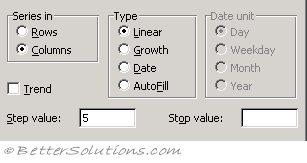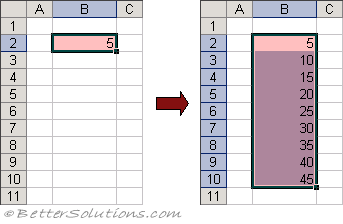# Linear Trends

In a linear series each cell differs from the previous cell by a fixed amount.
Adds the "step" value to the starting value and each cell in turnLinear - Creates a linear series or linear best-fit trend. This is selected by default. If the Trend box is cleared, a linear series is calculated by adding the value in the Step value box to each cell value in turn. If the Trend box is selected, the value in the Step value box is ignored, and a linear trend is calculated based on the selected values. The selected original values are replaced with values that fit the trend. This is covered in more detail on a subsequent page.
Trend - Calculates a best-fit line using the values that appear at the top or to the left of the selection. Any value in the Step value box is ignored if the Trend check box is selected. Excel will calculate the average change between the values already entered and uses that information to calculate a series to populate the remaining cells.